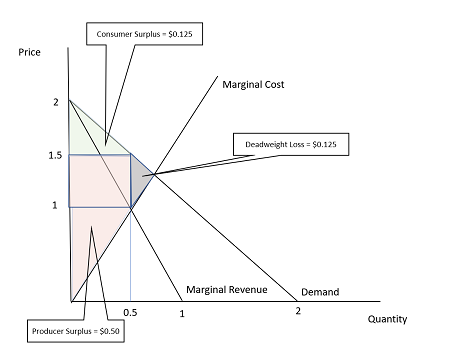# Suppose that a monopolist faces the demand curve P) 2 Q, and has total cost curve TC(Q) = Q^2. ...

## Question:

Suppose that a monopolist faces the demand curve P) 2 Q, and has total cost curve {eq}TC(Q) = Q^2. {/eq}

(a) If the firm is unable to price discriminate, find the firm's profit maximizing price and quantity.

(b) On a graph with quantity on the x-axis and price on the y-axis, plot the firm's marginal cost curve, marginal revenue curve, and the demand curve. Indicate the firm's profit maximizing price and quantity. Additionally, identify the areas on the graph that correspond to the consumer surplus, producer surplus, and deadweight loss

(c) Short answer: What is the source of deadweight loss in this market?

(d) Find the consumer surplus and producer surplus in the market.

## Price Discrimination:

Price discrimination is the (legal) process of charging different prices to different consumers based on their willingness to pay. With price discrimination, higher prices are charged to those who are willing to pay more while lower prices are charged to those who are not willing to pay as much.

(a) If the firm is unable to price discriminate, find the firm's profit maximizing price and quantity. The profit-maximizing price and output level are $1.50 and 0.5 units, respectively. If the firm is unable to price discriminate, it will produce at the output level where the marginal cost (MC) equals the marginal revenue (MR). They will then set the price such that the quantity demanded equals the profit-maximizing output level. When the demand curve is linear, the marginal revenue is equal to the demand curve, but double the slope, while the marginal cost is equal to the slope of the total cost curve, which can be found by solving for it's first derivative. {eq}P = 2 - Q\\ so\\ MR = 2 - 2Q\\ and\\ TC = Q^2\\ so\\ MC = 2Q therefore\\ MR = MC\\ 2 - 2Q = 2Q\\ 4Q = 2\\ Q = \frac{1}{2}\\ and\\ P = 2 - (\frac{1}{2})\\ P = 1.5 {/eq} (b) On a graph with quantity on the x-axis and price on the y-axis, plot the firm's marginal cost curve, marginal revenue curve, and the demand curve. Indicate the firm's profit maximizing price and quantity. Additionally, identify the areas on the graph that correspond to the consumer surplus, producer surplus, and deadweight loss. See below. The figure below illustrates all of these points/areas. The consumer surplus is the area below the demand curve and above the price, up to the quantity purchased while the producer surplus is the area above supply, below price, up to the quantity sold. The deadweight loss is the area between supply and demand between the quantity that was sold and the quantity where supply and demand equal each other.(c) Short answer: What is the source of deadweight loss in this market? Total surplus (consumer and producer surplus) is maximized where supply and demand equal each other. Because fewer units are produced and sold as a result of the monopoly, the deadweight loss occurs because of the lower production. (d) Find the consumer surplus and producer surplus in the market. The consumer and producer surpluses are$0.125 and \$0.50, respectively. As seen in the figure, the consumer surplus is the area of a triangle, which has an area equal to 0.5 times the base of the triangle times its height. The producer surplus is a triangle and a square, where the area of a square is equal to the base time the height.